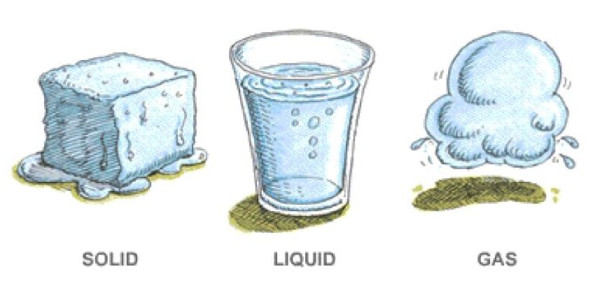# Properties Of Water: Trivia Quiz!

20 Questions | Total Attempts: 37Settings.

• 1.
Water covers over ........... of the earth’s surface.
• A.

90%

• B.

80%

• C.

70%

• D.

60%

• 2.
Water is used in many domestic activities such as....................
• 3.
Sources of water include ..................., .............., and ............
• 4.
Why is water considered to be a universal solvent?
• A.

Any substances dissolve in it

• B.

It is very acidi

• C.

It freezes quickly

• D.

All of the choices

• 5.
Which substance cannot dissolve in water?
• A.

Oil

• B.

Salt

• C.

Sugar

• D.

None of the choices

• 6.
What is the phase of water when its temperature is 150°C?
• A.

Solid

• B.

Liquid

• C.

Gas

• D.

None of the above

• 7.
Nearby water molecules are attracted to each other by:
• A.

Covalent bonds

• B.

Dipole bonds

• C.

Hydrogen bonds

• D.

Ionic bonds

• 8.
Molten lava pours from a volcano in Hawaii. After a few hours, the liquid lava hardens into a shiny gray rock. Which phase change has taken place?
• A.

Condensation

• B.

Freezing

• C.

Melting

• D.

Boiling

• 9.
An unopened bottle of water is taken out of the refrigerator and placed on a table. After a while, the bottle is covered with droplets of water. What phase change has occurred?
• A.

Melting

• B.

Freezing

• C.

Boiling

• D.

Condensation

• 10.
What phases are shown in the three images below?
• A.

Image 1 is solid, image 2 is gas, and image 3 is liquid.

• B.

Image 1 is gas, image 2 is solid, and image 3 is liquid.

• C.

Image 1 is gas, image 2 is liquid, and image 3 is solid.

• D.

Image 1 is liquid, image 2 is solid, and image 3 is gas.

• 11.
Boiling
• A.

Hydrogen and sodium

• B.

Hydrogen and oxygen

• C.

Hydrogen and oxygen

• 12.
How long can the average human survive without water?
• A.

How long can the average human survive without water?

• B.

A few weeks

• C.

A month

• D.

1 day

• 13.
At what temperature does water boil?
• A.

200 C

• B.

150 C

• C.

100 C

• D.

50 C

• 14.
At what temperature does water freeze?
• A.

-10 C

• B.

0 C

• C.

5 C

• D.

10 C

• 15.
What is the average pH level of pure water?
• A.

3

• B.

5

• C.

7

• D.

9

• 16.
In which step of the water cycle does liquid water transform into gas?
• A.

Transpiration

• B.

Condensation

• C.

Precipitation

• D.

Evaporation

• 17.
Which of the following is the chemical formula for water?
• A.

NaCl

• B.

H2O

• C.

CO2

• D.

W

• 18.
Which of the following is the solid state of water?
• A.

Ice

• B.

Liquid

• C.

Vapor

• D.

Solid

• 19.
What is 'potable' water?
• A.

• B.

Water that has been polluted

• C.

Water that is drinkable

• D.

Salt water from the oceans

• 20.
Water is only found on Earth because Hydrogen and Oxygen are rarely found outside the Earth's atmosphere.
• A.

True

• B.

False

Related TopicsBack to top